ESTIMATE Statement
ESTIMATE ’label’ effect values <...effect values> </ options> ;

The ESTIMATE statement enables you to estimate linear functions of the parameters by multiplying the vectorby the parameter estimate vector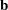, resulting in. All of the elements of thevector might be given, or, if only certain portions of thevector are given, the remaining elements are constructed by PROC GLM from the context (in a manner similar to rule 4 discussed in the section Construction of Least Squares Means).

The linear function is checked for estimability. The estimate, where, is displayed along with its associated standard error,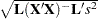, andtest. If you specify the CLPARM option in the MODEL statement, confidence limits for the true value are also displayed.

There is no limit to the number of ESTIMATE statements that you can specify, but they must appear after the MODEL statement. In the ESTIMATE statement,

label

identifies the estimate on the output. A label is required for every contrast specified. Labels must be enclosed in quotes.

effect

identifies an effect that appears in the MODEL statement, or the INTERCEPT effect. The INTERCEPT effect can be used as an effect when an intercept is fitted in the model. You do not need to include all effects that are in the MODEL statement.

values

are constants that are the elements of thevector associated with the preceding effect. For example,

```estimate 'A1 VS A2' A  1 -1;
```

forms an estimate that is the difference between the parameters estimated for the first and second levels of the CLASS variable A.

You can specify the following options in the ESTIMATE statement after a slash (/):

DIVISOR=number

specifies a value by which to divide all coefficients so that fractional coefficients can be entered as integer numerators. For example, you can use

```estimate '1/3(A1+A2) - 2/3A3' a 1 1 -2 / divisor=3;
```

```estimate '1/3(A1+A2) - 2/3A3' a 0.33333 0.33333 -0.66667;
displays the entirevector. This option is useful in confirming the ordering of parameters for specifying.
tunes the estimability checking. If ABS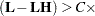number, then thevector is declared nonestimable.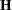is the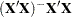matrix, andis ABSexcept for rows whereis zero, and then it is 1. The default value for the SINGULAR= option is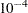. Values for the SINGULAR= option must be between 0 and 1.